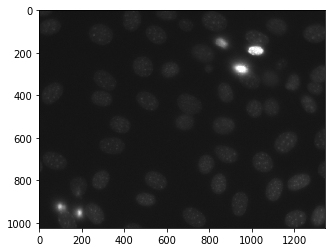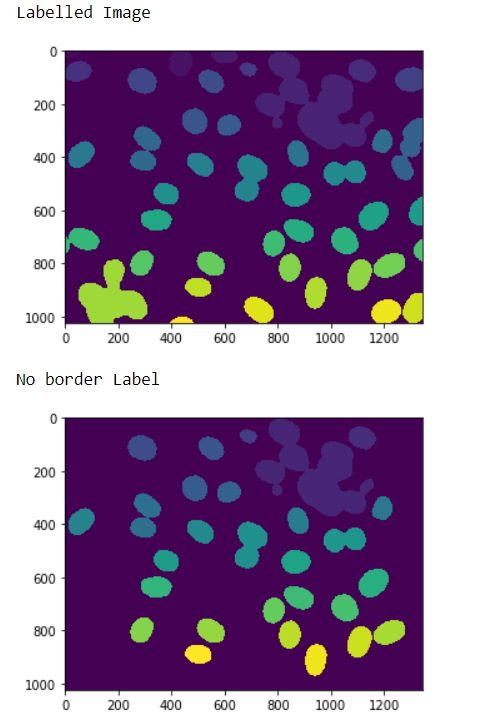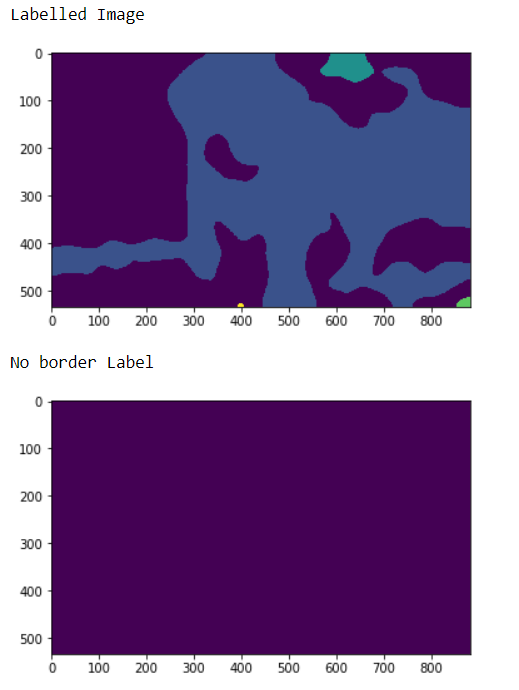# Mahotas – Removing Bordered Labeled

In this article we will see how we can remove the bordered label from the labelled image in mahotas. Border labels are those labels which are touching the border, we can create labelled image from normal image with the help of `mahotas.label` method.

For this we are going to use the fluorescent microscopy image from a nuclear segmentation benchmark. We can get the image with the help of command given below

`mhotas.demos.nuclear_image()`

Below is the nuclear_imageLabeled images are integer images where the values correspond to different regions. I.e., region 1 is all of the pixels which have value 1, region two is the pixels with value 2, and so on

In order to do this we will use `mahotas.remove_bordering` method

Syntax : mahotas.remove_bordering(labeled)

Argument : It takes labeled image object as argument

Return : It returns the labelled image without label at the borders

Example 1:

 `# importing required libraries ` `import` `mahotas ` `import` `numpy as np ` `from` `pylab ``import` `imshow, show ` `import` `os ` ` `  `# loading nuclear image ` `f ``=` `mahotas.demos.load(``'nuclear'``) ` ` `  `# setting filter to the image ` `f ``=` `f[:, :, ``0``] ` ` `  `# setting gaussian filter ` `f ``=` `mahotas.gaussian_filter(f, ``4``) ` ` `  `# setting threshold value ` `f ``=` `(f> f.mean()) ` ` `  `# creating a labeled image ` `labeled, n_nucleus ``=` `mahotas.label(f) ` ` `  `# showing the labeleed image ` `print``(``"Labelled Image"``) ` `imshow(labeled) ` `show() ` ` `  `# removing border labels ` `labeled ``=` `mahotas.labeled.remove_bordering(labeled) ` ` `  `# showing the image ` `print``(``"No border Label"``) ` `imshow(labeled) ` `show() `

Output :Example 2:

 `# importing required libraries ` `import` `numpy as np ` `import` `mahotas ` `from` `pylab ``import` `imshow, show ` ` `  `# loading iamge ` `img ``=` `mahotas.imread(``'dog_image.png'``) ` `   `  `# filtering the imagwe ` `img ``=` `img[:, :, ``0``] ` `    `  `# setting gaussian filter ` `gaussian ``=` `mahotas.gaussian_filter(img, ``15``) ` ` `  `# setting threshold value ` `gaussian ``=` `(gaussian > gaussian.mean()) ` ` `  `# creating a labeled image ` `labeled, n_nucleus ``=` `mahotas.label(gaussian) ` `  `  `print``(``"Labelled Image"``) ` `# showing the gaussian filter ` `imshow(labeled) ` `show() ` ` `  `# removing border labels ` `labeled ``=` `mahotas.labeled.remove_bordering(labeled) ` ` `  `# showing the image ` `print``(``"No border Label"``) ` `imshow(labeled) ` `show() `

Output :My Personal Notes arrow_drop_upCheck out this Author's contributed articles.

If you like GeeksforGeeks and would like to contribute, you can also write an article using contribute.geeksforgeeks.org or mail your article to contribute@geeksforgeeks.org. See your article appearing on the GeeksforGeeks main page and help other Geeks.

Please Improve this article if you find anything incorrect by clicking on the "Improve Article" button below.

Article Tags :

Be the First to upvote.

Please write to us at contribute@geeksforgeeks.org to report any issue with the above content.